# do the 4th question plz 1-(25%) Draw shear and moment diagrams for the beam shown in Figure P-1. Draw a sketch of the deflected shape 2-(25%) Using moment area method, for the beam shown in Figure...

do the 4th question plz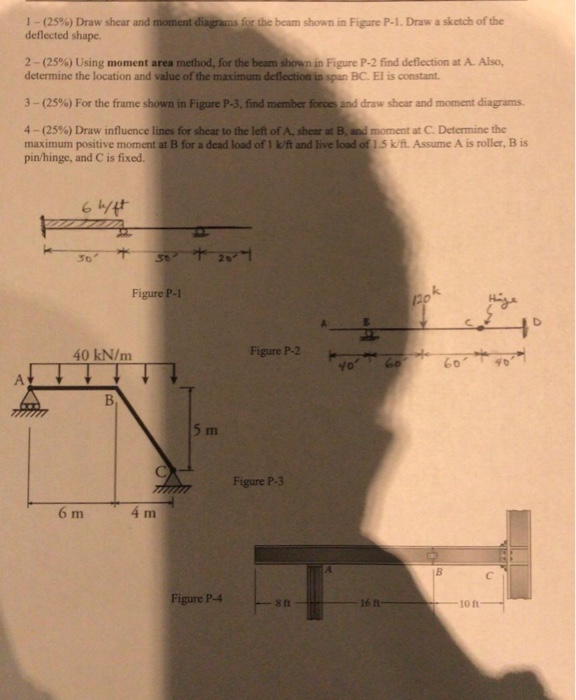1-(25%) Draw shear and moment diagrams for the beam shown in Figure P-1. Draw a sketch of the deflected shape 2-(25%) Using moment area method, for the beam shown in Figure P-2 find deflection at A. Also, determine the location and value of the maximum deflection in span BC. El is constant 3-(25%) For the frame shown in Figure P-3-find member frees and draw shear and moment diagrams. 4-(25%) Draw/ influence lines for shear to the left ofA, shear at B, and moment at C. Determine the maximum positive moment at B for a dead load of 1 k/ft and live load of 15k/ft. Assume A is roller, B is pin/hinge, and C is fixed. 50 k. 40 kN/m gure P mFigure P-3 6 m Figure P-4 16n 10 n 8 ft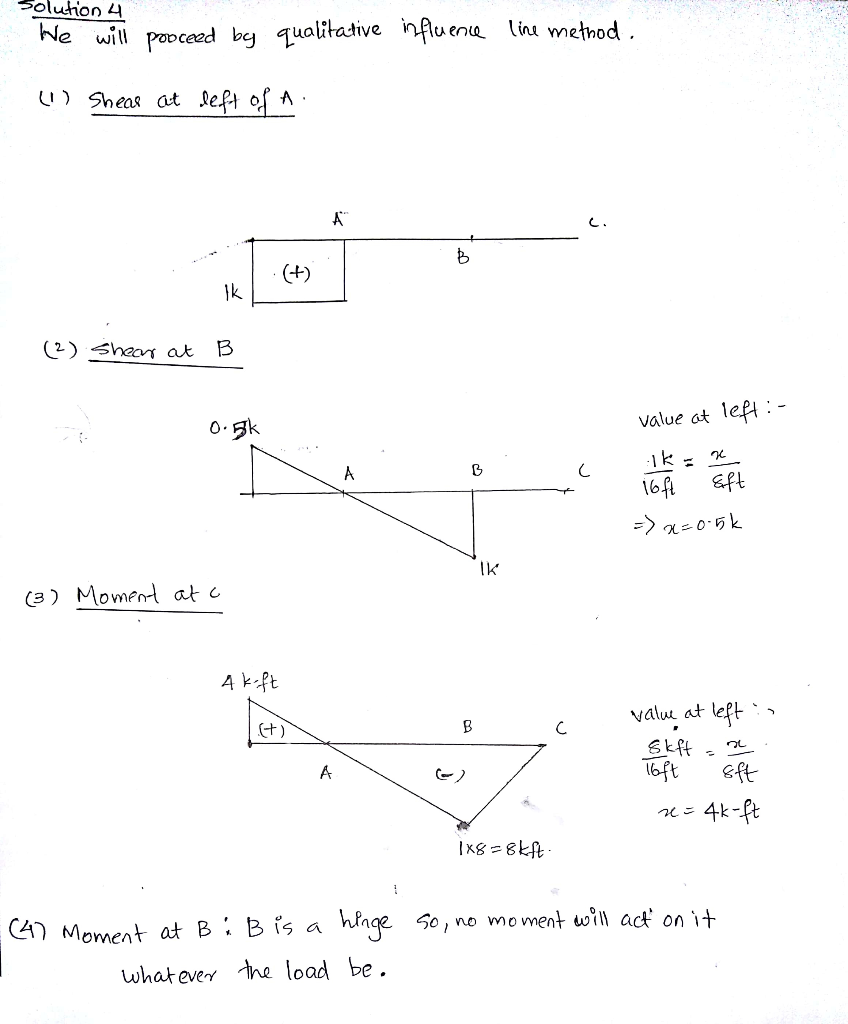#### Earn Coin

Coins can be redeemed for fabulous gifts.

Similar Homework Help Questions
• ### 3 plz Final 1-(25%) Draw shear and moment diagrams for the beam shown in Figure P-1....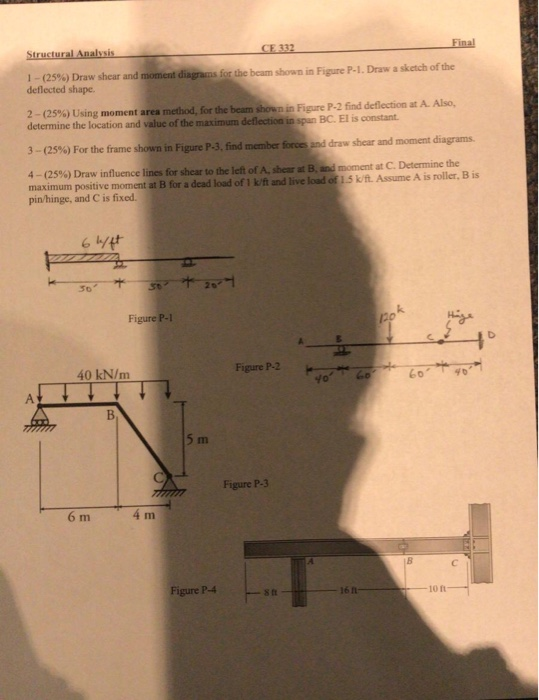3 plz Final 1-(25%) Draw shear and moment diagrams for the beam shown in Figure P-1. Draw a sketh of the deflected shape. 2-125%) Using moment area method, for the beams os Figure P 2 find deflection at A Also, determine the location and valuc of the maximum deflection in span BC. El is constant d draw shear and moment diagrams. 3-(25%) For the frame shown in Figure P-3, find member fr e moment at C. Determine the 4-(25%) Draw...

• ### 1-(25%) Draw shear and moment diagrams for the beam shown in Figure P-1 . Draw a sketch of the deflected shape. The spans are 30-ft long each (total of 90-ft), the concentrated loads are eac...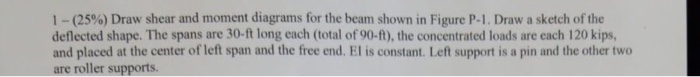1-(25%) Draw shear and moment diagrams for the beam shown in Figure P-1 . Draw a sketch of the deflected shape. The spans are 30-ft long each (total of 90-ft), the concentrated loads are each 120 kips, and placed at the center of left span and the free end. El is constant. Left support is a pin and the other two are roller supports. 120 kips 120 kips Figure P-1 1-(25%) Draw shear and moment diagrams for the beam shown...

• ### Question 2. Draw the shear and bending-moment diagrams for the beam and loading shown, and determine...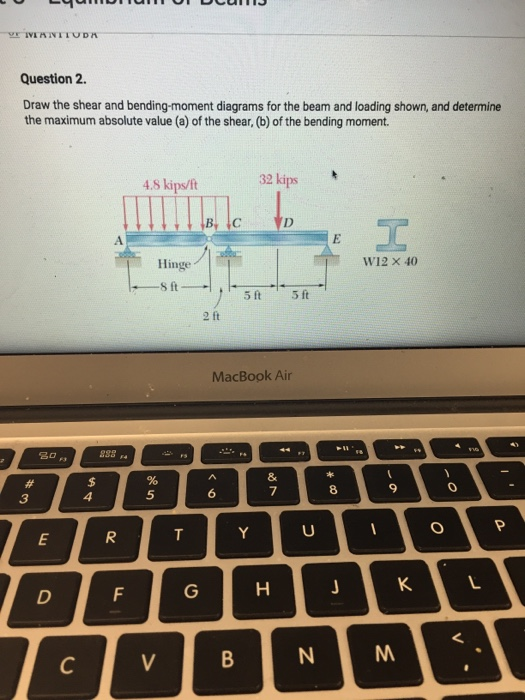Question 2. Draw the shear and bending-moment diagrams for the beam and loading shown, and determine the maximum absolute value (a) of the shear, (b) of the bending moment. 4.8 kips/ft 32 kips Hinge W12 × 40 5ft 5 ft 2 ft MacBook Air 838 a 5

• ### Please explain Number 3. 2 - Using moment area method, for the beam shown in Figure P-2 find deflection at the center (point C) and rotation under the concentrated load (point D). Also, find locat...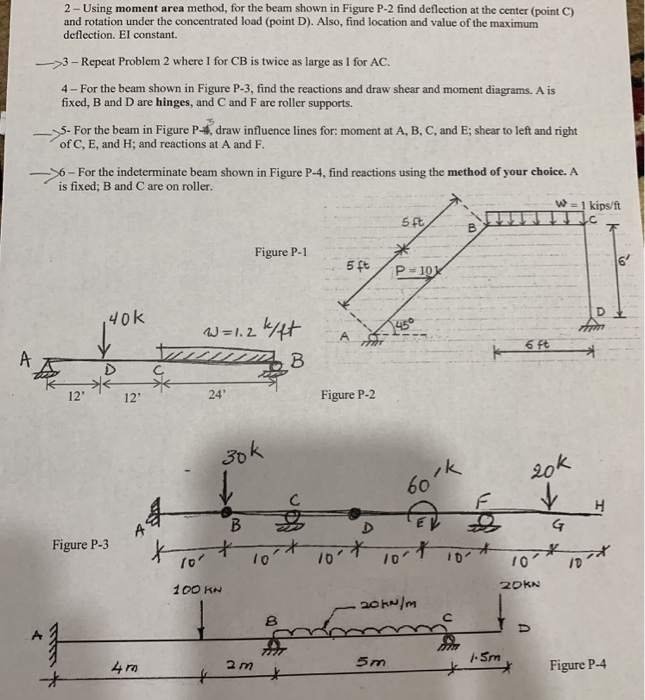Please explain Number 3. 2 - Using moment area method, for the beam shown in Figure P-2 find deflection at the center (point C) and rotation under the concentrated load (point D). Also, find location and value of the maximunm deflection. EI constant. 3- Repeat Problem 2 where I for CB is twice as large as I for AC. 4 - For the beam shown in Figure P-3, find the reactions and draw shear and moment diagrams. A is fixed,...

• ### Draw the Shear & Moment Diagrams and sketch the deflected shape for the beams shown below...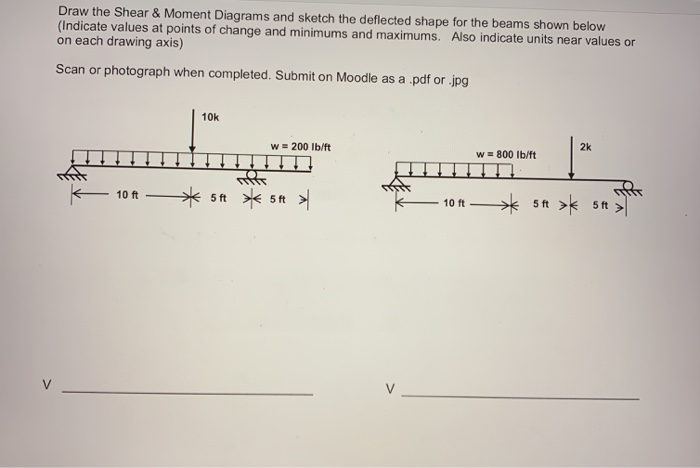Draw the Shear & Moment Diagrams and sketch the deflected shape for the beams shown below (Indicate values at points of change and minimums and maximums. Also indicate units near values or on each drawing axis) Scan or photograph when completed. Submit on Moodle as a .pdf or .jpg 10k w = 200 lb/ft 2k W = 800 lb/ft 10 ft 5 ft 5ft > 10 ft ** 5+ > 5 > M M D D

• ### Analyze the Frames shown below. Draw the shear and moment diagrams and sketch the approximate deflected...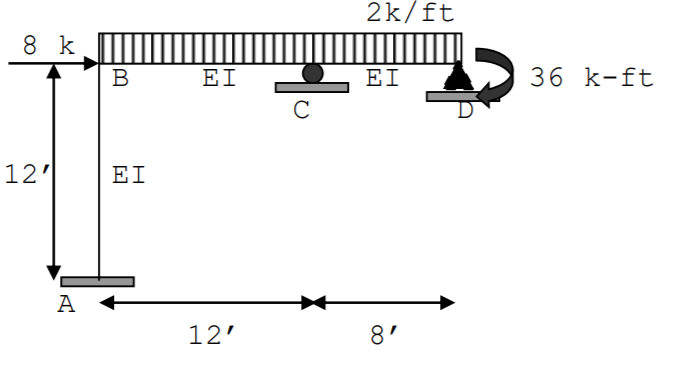Analyze the Frames shown below. Draw the shear and moment diagrams and sketch the approximate deflected shape Settlement at A = 1” and at C = .5” E = 30000 kis, I = 240 in4 2k/ft 8 k B EI EI 36 k-ft С 12'1 EI A 12' 8'

• ### SHEAR AND MOMENT DIAGRAMS Determine the shear and moment diagrams for the beam shown below. There...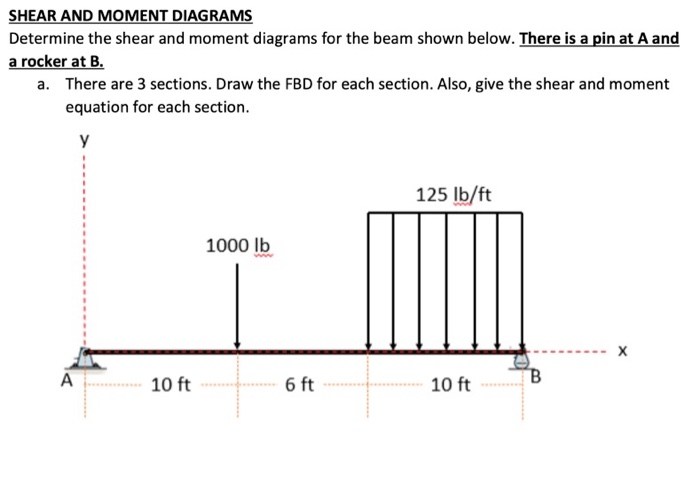SHEAR AND MOMENT DIAGRAMS Determine the shear and moment diagrams for the beam shown below. There is a pin at A and a rocker at B. a. There are 3 sections. Draw the FBD for each section. Also, give the shear and moment equation for each section. у 125 lb/ft 1000 lb A 10 ft 6 ft 10 ft B

• ### A) The internal span CD on a continuous beam is deflected as shown (Figure 2) as...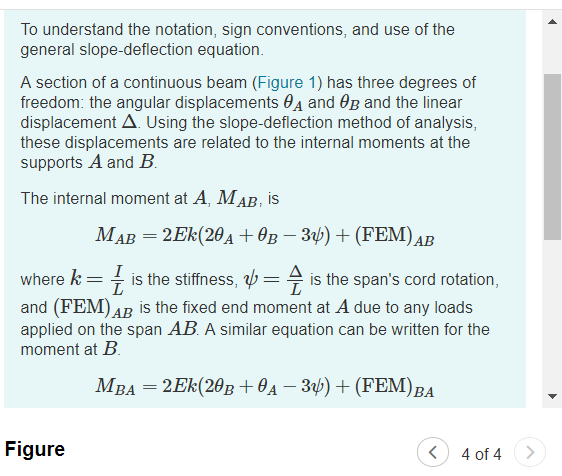A) The internal span CD on a continuous beam is deflected as shown (Figure 2) as a result of loads on other portions of the beam, where L = 25 ft , α = 0.75 ∘, β = 0.35 ∘, E = 2.9×104 ksi , and I = 1500 in4 . There is no vertical deflection at either end. What is the internal moment at D? M_D B)The end span EF on a continuous beam is deflected as shown (Figure...

• ### A simply supported beam as shown in the figure. The beam section is W18x211. The beam...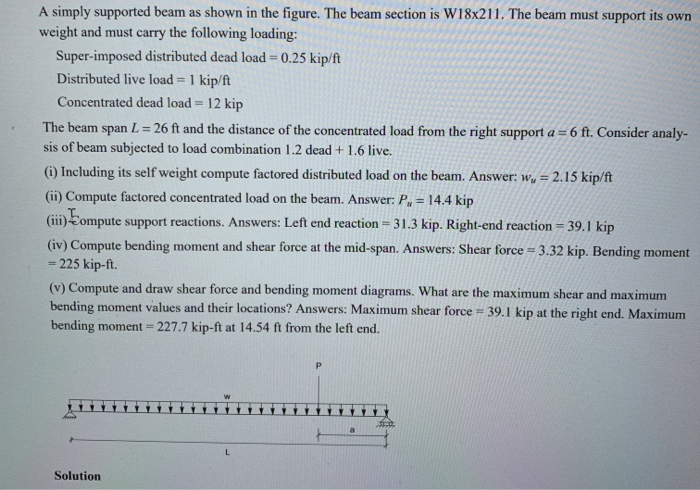A simply supported beam as shown in the figure. The beam section is W18x211. The beam must support its own weight and must carry the following loading: Super-imposed distributed dead load = 0.25 kip/ft Distributed live load = 1 kip/ft Concentrated dead load = 12 kip The beam span L = 26 ft and the distance of the concentrated load from the right support a=6 ft. Consider analy- sis of beam subjected to load combination 1.2 dead + 1.6 live....

• ### 4. SHEAR AND MOMENT DIAGRAMS Determine the shear and moment diagrams for the beam shown below....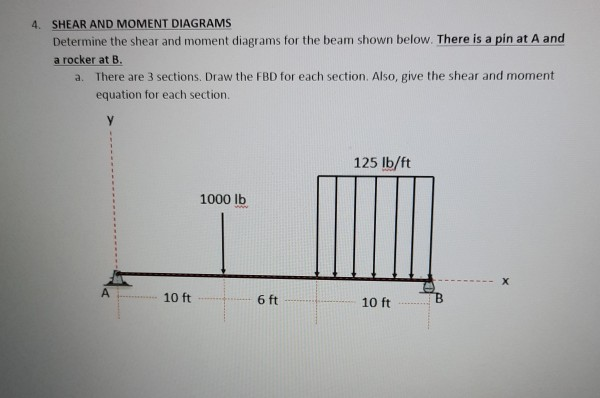4. SHEAR AND MOMENT DIAGRAMS Determine the shear and moment diagrams for the beam shown below. There is a pin at A and a rocker at B. a. There are 3 sections. Draw the FBD for each section. Also, give the shear and moment equation for each section. 125 lb/ft 1000 lb х 10 ft 6 ft 10 ft

Free Homework Help App